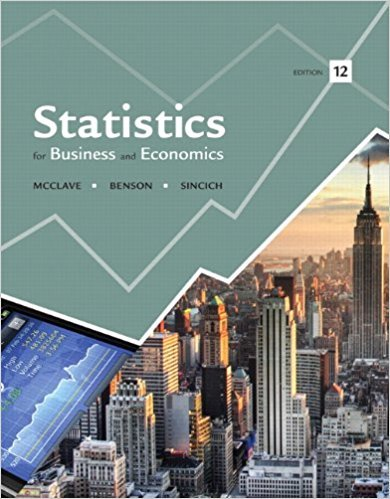×
×

# Solutions for Chapter 2: Statistics for Business and Economics 12th Edition## Full solutions for Statistics for Business and Economics | 12th Edition

ISBN: 9780321826237Solutions for Chapter 2

Solutions for Chapter 2
4 5 0 323 Reviews
27
1
##### ISBN: 9780321826237

Since 171 problems in chapter 2 have been answered, more than 99259 students have viewed full step-by-step solutions from this chapter. This expansive textbook survival guide covers the following chapters and their solutions. This textbook survival guide was created for the textbook: Statistics for Business and Economics , edition: 12. Chapter 2 includes 171 full step-by-step solutions. Statistics for Business and Economics was written by and is associated to the ISBN: 9780321826237.

Key Business Terms and definitions covered in this textbook
• accounting profit

total revenue minus total explicit cost

the tendency for the mix of unobserved attributes to become undesirable from the standpoint of an uninformed party

• club goods

goods that are excludable but not rival in consumption

the ability to produce a good at a lower opportunity cost than another producer

• constant returns to scale

The property whereby long-run average total cost stays the same as the quantity of output changes

• cost

the value of everything a seller must give up to produce a good

• cost–benefit analysis

a study that compares the costs and benefits to society of providing a public good

• cross-price elasticity of demand

a measure of how much the quantity demanded of one good responds to a change in the price of another good, computed as the percentage change in quantity demanded of the first good divided by the percentage change in price of the second good

• diminishing returns

the property whereby the benefit from an extra unit of an input declines as the quantity of the input increases

• efficiency wages

above-equilibrium wages paid by firms to increase worker productivity

• equilibrium

a situation in which the market price has reached the level at which quantity supplied equals quantity demanded

• equilibrium price

the price that balances quantity supplied and quantity demanded

• factors of production

the inputs used to produce goods and services

• inflation rate

the percentage change in the price index from the preceding period

• marginal cost

the increase in total cost that arises from an extra unit of production

• price elasticity of supply

a measure of how much the quantity supplied of a good responds to a change in the price of that good, computed as the percentage change in quantity supplied divided by the percentage change in price

• quantity demanded

the amount of a good that buyers are willing and able to purchase

• surplus

a situation in which quantity supplied is greater than quantity demanded

• unit of account

the yardstick people use to post prices and record debts

• variable costs

costs that vary with the quantity of output produced

×